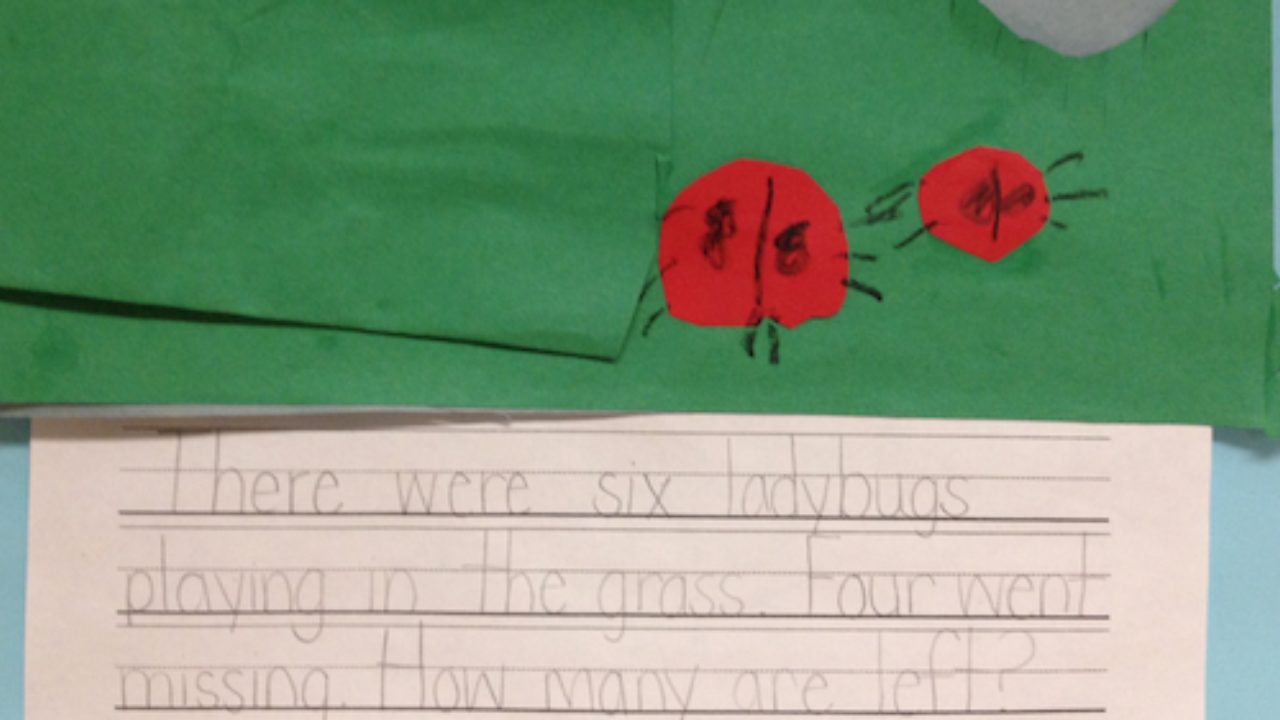# Subtraction Strategies - Comparison Method

May 05, 2022Often when we are talking with young children about subtraction we use the term “take away.”  While we can sometimes think of subtraction as taking away, subtraction can also mean finding the difference between two numbers.  Knowing multiple subtraction strategies helps us pick the best strategy for the situation.  Let’s take a look.

When we have a problem like:

There were eight birds, four flew away.  How many are left?

This problem can easily be discussed by the language of taking some of the birds away.

Consider, however, a problem such as this:

Sam is 46 inches tall. Hannah is 39 inches tall.  How much taller is Sam?

In this instance, we are comparing two quantities and cannot take something away in order to find the answer.

## Modeling with the Comparison Method

When we cannot easily take some away, the comparison method works is effective for finding the answer.  We compare two quantities and see what the difference is between the two.  For smaller numbers, unifix cubes are used for modeling the comparison method.  Students make two trains – one for each number – and then compare their lengths.  They can easily count how many more cubes are in one train than the other.  Here I have modeled 11-7.  Do you see the space between the two which has a size of 4?

As the numbers get bigger, Base Ten Blocks are a great tool to use when exploring the comparison method.  Students can easily build one number and then build the second number on top of the first number.  The cubes that do not have an extra layer on top represent the difference.  My second graders liked to ask, “How many are not double stacked?”  Here I have modeled 42-27.  First we build the 42.  Then we stack the 27 on top.  The ones that are still showing blue show the difference between the blue group of 42 and the yellow group of 27.

I once worked with an adult who claimed that one could not find the difference of 27 and 42 because, “You cannot take a larger number from a smaller number.”  This is the difficulty that can arise when students are only thinking about taking away and are not thinking about subtraction as comparing two quantities.

## Part-Whole Subtraction Problems

We can also use the comparison method for a problem where we know the size of the whole but don’t know the size of one of the parts. Consider a problem like:I have 14 jelly beans.  8 are red.  How many are not red?Practice some of these different types of problems with your child using unifix cubes, base ten blocks, or other counters (pennies, cheerios, goldfish crackers, buttons, etc.) you have at home.  Then present some problems and discuss what strategy would work best for that problem.  Is it a take-away problem or a difference problem?  MP # 5 calls for students to “Use appropriate tools strategically.”  By knowing multiple subtraction strategies, your child can pick the best one for the situation and work with understanding.  If you would like to learn more about subtraction strategies, you can take my online course for parents of 2nd-5th grade parents.